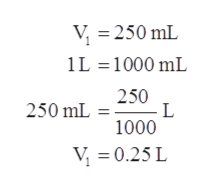# The gas in a 250. mL piston experiences a change in pressure from 1.00 atm to 4.75 atm. What is the new volume (in mL) assuming the moles of gas and temperature are held constant?

Question
14 views

The gas in a 250. mL piston experiences a change in pressure from 1.00 atm to 4.75 atm. What is the new volume (in mL) assuming the moles of gas and temperature are held constant?

check_circle

Step 1

Given information:

Pressure of the gas at initial stage (P1) = 1.00 atm

Volume of the sample at initial stage (V1) = 250 mL

Pressure of the gas at final stage (P2) = 4.75 atm

Step 2

Bolye’s law states that the pressure of an ideal gas is inversely proportional to its volume. The expression is given as follows:

Step 3

As volume is given in mL so it should be ...help_outlineImage TranscriptioncloseV = 250 mL 1L = 1000 mL 250 250 mL = . 1000 V, = 0.25 L fullscreen

### Want to see the full answer?

See Solution

#### Want to see this answer and more?

Solutions are written by subject experts who are available 24/7. Questions are typically answered within 1 hour.*

See Solution
*Response times may vary by subject and question.
Tagged in
ScienceChemistry

### Gas laws